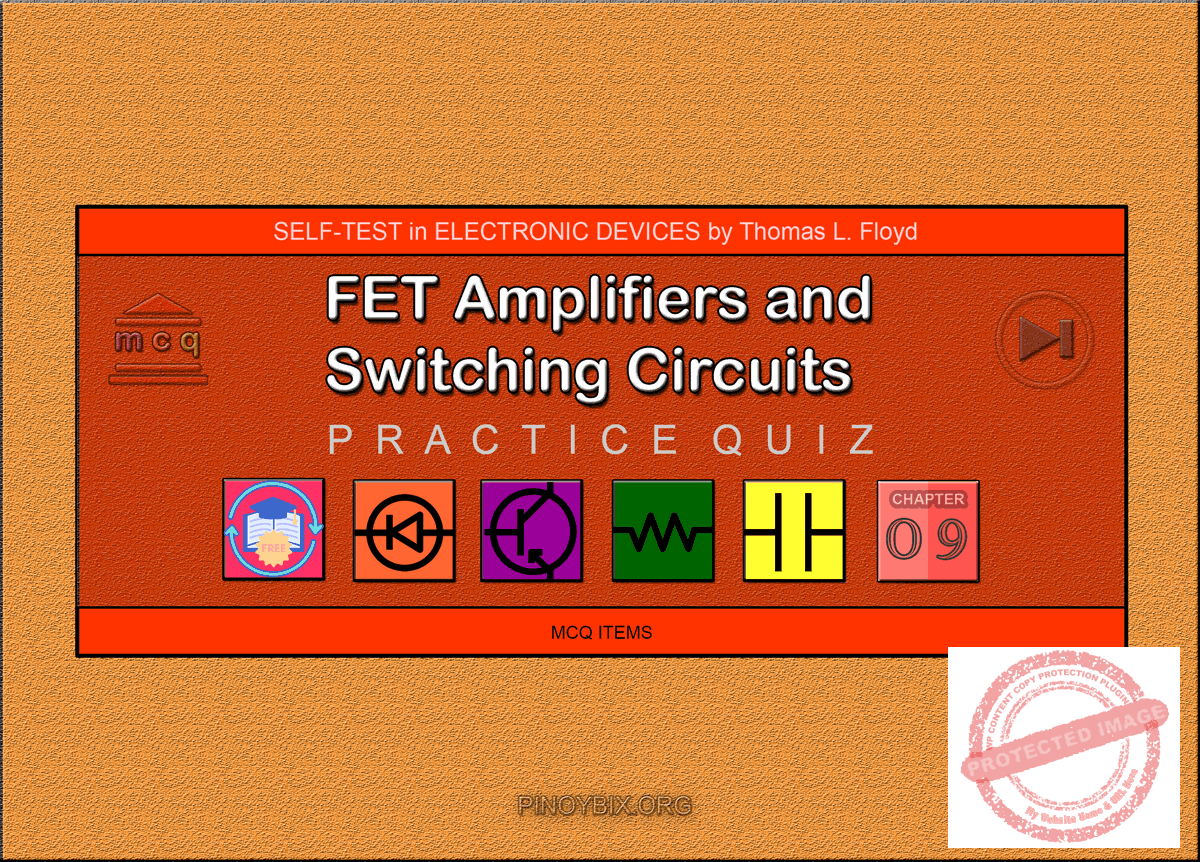# Floyd Self-test in FET Amplifiers and Switching Circuits

(Last Updated On: December 5, 2019)This is the Self-test in Chapter 9: FET Amplifiers and Switching Circuits from the book Electronic Devices Conventional Current Version, 9th edition by Thomas L. Floyd. If you are looking for a reviewer in Electronics Engineering this will definitely help you before taking the Board Exam.

#### Floyd Self-test Chapter 9 Topic Outline

• Floyd Self-test in The Common-Source Amplifier
• Floyd Self-test in The Common-Drain Amplifier
• Floyd Self-test in The Common-Gate Amplifier
• Floyd Self-test in The Class D Amplifier
• Floyd Self-test in MOSFET Analog Switching
• Floyd Self-test in MOSFET Digital Switching

If you are looking for the Multiple Choice Questions in Floyd’s Electronic Devices proceed to

#### Start Practice Exam Test Questions

Choose the letter of the best answer in each questions.

1. In a common-source amplifier, the output voltage is

(a) 180ᵒ out of phase with the input

(b) in phase with the input

(c) taken at the source

d) taken at the drain

(f ) answers (a) and (d)

Solution:

2. In a certain common-source (CS) amplifier, Vds= 3.2 Vrms and Vgs =280 mVrms. The voltage gain is

(a) 1

(b) 11.4

(c) 8.75

(d) 3.2

Solution:

3. In a certain CS amplifier, RD = 1.0kΩ,RS = 560Ω, VDD = 10 V, AND gm = 4500 μS If the source resistor is completely bypassed, the voltage gain is

(a) 450

(b) 45

(c) 4.5

(d) 2.52

Solution:

4. Ideally, the equivalent circuit of a FET contains

(a) a current source in series with a resistance

(b) a resistance between drain and source terminals

(c) a current source between gate and source terminals

(d) a current source between drain and source terminals

Solution:

5. The value of the current source in Question 4 is dependent on the

(a) transconductance and gate-to-source voltage

(b) dc supply voltage

(c) external drain resistance

Solution:

6. A certain common-source amplifier has a voltage gain of 10. If the source bypass capacitor is removed,

(a) the voltage gain will increase

(b) the transconductance will increase

(c) the voltage gain will decrease

(d) the Q-point will shift

Solution:

7. A CS amplifier has a load resistance of 10 kΩ and RD = 820Ω. If gm = 5 mS and Vin = 500 mV, the output signal voltage is

(a) 1.89 V

(b) 2.05 V

(c) 25 V

(d) 0.5 V

Solution:

8. If the load resistance in Question 7 is removed, the output voltage will

(a) stay the same

(b) decrease

(c) increase

(d) be zero

Solution:

9. A certain common-drain (CD) amplifier with RS= 1.0 kΩ has a transconductance of 6000 μS .The voltage gain is

(a) 1

(b) 0.86

c) 0.98

(d) 6

Solution:

10. The datasheet for the transistor used in a CD amplifier specifies IGSS = 5 nA at VGS = 10 V. If the resistor from gate to ground, RG, is 50MΩ, the total input resistance is approximately

(a) 50MΩ

(b) 200MΩ

(c) 40MΩ

(d) 20.5MΩ

Solution:

11. The common-gate (CG) amplifier differs from both the CS and CD configurations in that it has a

(a) much higher voltage gain

(b) much lower voltage gain

(c) much higher input resistance

(d) much lower input resistance

Solution:

12. If you are looking for both good voltage gain and high input resistance, you must use a

(a) CS amplifier

(b) CD amplifier

(c) CG amplifier

Solution:

13. A cascode amplifier consists of

(a) a CD and a CS amplifier

(b) a CS and a CG amplifier

(c) a CG and a CD amplifier

(d) two CG amplifiers

Solution:

14. The class D amplifier is similar to

(a) class C

(b) class B

(c) class A

(d) none of these

Solution:

15. The class D amplifier uses

(a) frequency modulation

(b) amplitude modulation

(c) pulse-width modulation

(d) duty cycle modulation

Solution:

16. E-MOSFETs are generally used for switching applications because of their

(a) threshold characteristic

(b) high input resistance

(c) linearity

(d) high gain

Solution:

17. A sampling circuit must sample a signal at a minimum of

(a) one time per cycle

(b) the signal frequency

(c) twice the signal frequency

(d) alternate cycles

Solution:

18. The value of resistance emulated by a switched-capacitor circuit is a function of

(a) voltage and capacitance

(b) frequency and capacitance

(c) gain and transconductance

(d) frequency and transconductance

Solution:

19. A basic CMOS circuit uses a combination of

(a) n-channel MOSFETs

(b) p-channel MOSFETs

(c) pnp and npn BJTs

(d) an n-channel and a p-channel MOSFET

Solution:

20. CMOS is commonly used in

(a) digital circuits

(b) linear circuits

(c) RF circuits

(d) power circuits

Solution:

21. If there is an internal open between the drain and source in a CS amplifier, the drain voltage is equal to

(a) 0 V

(b) VDD

(c) VGS

(d) VGD

Solution:

### Complete List of Floyd Self-test in Electronic Devices

P inoyBIX educates thousands of reviewers and students a day in preparation for their board examinations. Also provides professionals with materials for their lectures and practice exams. Help me go forward with the same spirit.

“Will you subscribe today via YOUTUBE?”

Subscribe

PinoyBIX Engineering. © 2014-2020 All Rights Reserved | How to Donate? |